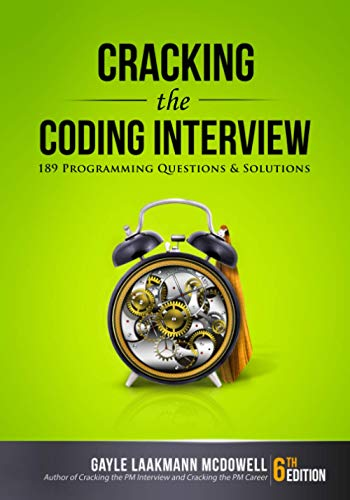# Number of Ones

Question: Write a function that determines the number of bits set to 1 in the binary representation of an integer.

Answer: Going by the brute force approach, it would be easy to come up with the following solution. If the least significant bit of a number is 1, it will return a result of 1 when we AND it with 1. Using this logic, we can shift bits in the number to the right and keep testing if the least significant bit is 1. When there are no 1’s left in the number, it will become zero. Here’s some code to illustrate this simple logic.

```int numOnesInInteger( int num )
{
int numOnes = 0;
while( num != 0 )
{
if( ( num & 1 ) == 1 ) {
numOnes++;
}
num = num >> 1;
}
return numOnes;
}
```

Think we can do better? The above algorithm runs in O(n) where n in the number of bits in the integer. Maybe we can make this a tad better. But this would need some real creative bit manipulation skills. So let’s think, what happens to a number when it we AND it with (number – 1). Let’s take 7 (0111) as an example.

7 & 6 = 0111 & 0110 = 0110 = 6
6 & 5 = 0110 & 0101 = 0100 = 4
4 & 3 = 0100 & 0011 = 0000 = 0

Do you see a pattern yet? Essentially, when you AND a number with (number – 1), the last 1 bit in the number gets set to zero. So if we continue to set every 1 bit in the number to zero, the number will eventually become zero. If we use this logic to count the number of 1s, we have an algorithm that runs in O(m) where m is the number of bits set to 1 in the number. Here’s some code.

```int numOnesInInteger( int num )
{
int numOnes = 0;
while( num != 0 ){
num = num & (num – 1);
numOnes++;
}
return numOnes;
}
```

Interesting right? Please Digg or Stumble this post! As usual, comments are always welcome.

If you're looking for some serious preparation for your interviews, I'd recommend this book written by a lead Google interviewer. It has 189 programming questions and solutions:## 9 Responses

1.satyam says:

int count_bits(unsigned int num){

int i =0,count=0;
while(i!=31){
if(num&(1<<i){
count ++;
}
i++;
}
return count;
}

2.Bill Strathearn says:

There is a bug in the code from YikeWang.

3.jagannath pattar says:

Its simple!!!

/*
* A program to count number of one’s in an integer.
* [email protected]
*/

#include

int number_of_ones(int num)
{
int i;
for(i = 0; num != 0; i++)
num = num & num – 1;
return i;
}

main()
{
int num, count = 0;
printf(“Enter the number: “);
scanf(“%d”,&num);
count = number_of_ones(num);
printf(“\nNumber of one’s are %d\n”, count);
}

4.vitaly says:

unsigned int val;
unsigned int cnt = ((val&0xAAAAAAAA)>>1) + (val&0x55555555);
cnt = ((cnt&0xCCCCCCCC)>>2) + (cnt&0x33333333);
cnt = ((cnt&0xF0F0F0F0)>>4) + (cnt&0x0f0f0f0f);
cnt = ((cnt&0xFF00FF00)>>8) + (cnt&0xFF00FF);
cnt = ((cnt&0xFFFF0000)>>16) + (cnt&0xFFFF);

5.Vishal says:

Approach 1 mentioned above is good only for unsigned integers, run it for num = -1 in Java and you will see an issue because Java does not have unsigned integers and >> will cause a sign bit shift causing issue. Fix is to use the unsigned right shift operator (>>>) in following line:

``` num = num >>> 1; ```

6.Janeev Joy says:

What is the last code snippet(@YikeWang) ??

7.YikeWang says:
``` int CountBits (unsigned int x )
{
static unsigned int mask[] = { 0x55555555,
0x33333333,
0x0F0F0F0F,
0x00FF00FF,
0x0000FFFF
} ;

int i ;
int shift ;
for (i =0, shift =1; i > shift) & mask[i]);
return x;
}
```
8.Aswin says:

You can represent any number in this format xxxxx100 (or xxxxxxx1 or xxxxxx10 or xxxx1000 and so on…) where x can be any bit.

n = xxxxx100 and n-1 = xxxxx011 => n & (n-1) = xxxxx000

Notice how anding the n and n-1 removed the right-most 1 bit. Each time we do n & (n-1), we remove the right-most 1 bit. If there are m bits set to 1, all the bits will be cleared after m iterations.

Is this any clearer? Let me know if this is still confusing.

9.Ashwin says:

Can you explain why the pattern is true for any two numbers n & n-1? What I mean is, why is it (can you prove) that for any two numbers n and n-1, the result of their AND operation will always result in the set bit in the least significant position set to zero?

XHTML: These are some of the tags you can use: `<a href=""> <b> <blockquote> <code> <em> <i> <strike> <strong>`